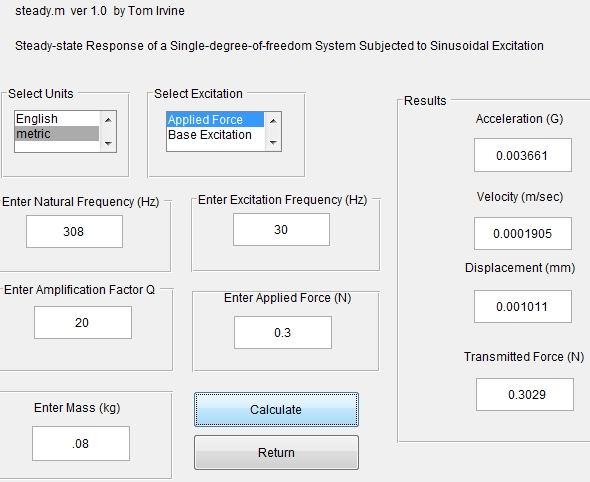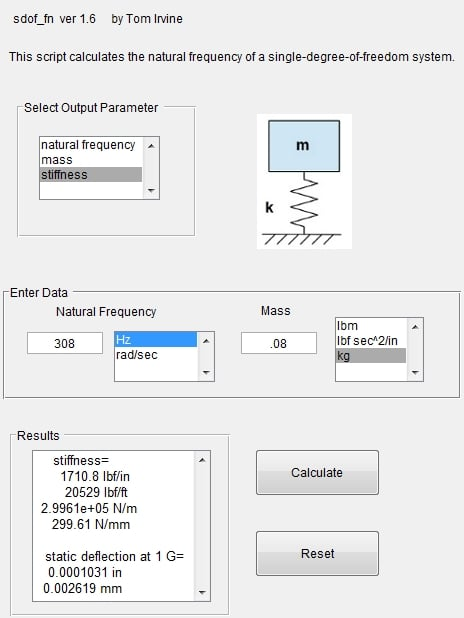peteroznewman
Subscriber

A Single Degree of Freedom (SDOF) system has a mass connected to ground through a spring. The spring-mass system has a natural frequency. If you study dynamics, you will find there is a standard equation to predict the motion of that SDOF system to sinusoidal input.  You gave me all the inputs I needed except for the mass, so I used 80 grams. If you have a sinusoidal force of 0.3 N acting on that mass, and the natural frequency is 308 Hz, the reaction force on the ground is going to be 0.303 N and the displacement is going to be 1 micron. I used matlab and a script written by Tom Irvine to solve the standard equations.Here is the spring stiffness needed to get 308 Hz with an 80 gram mass:At these low forcing frequencies, I don't think there will be much difference how many modes you include, but the cost of including them is low so put in 6.

I expect either way of obtaining the Reaction Force will work. If you do it both ways, do you get the same result?